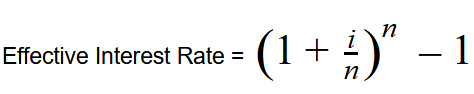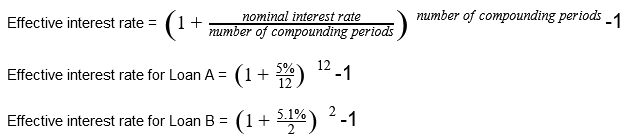# Effective Interest Rate

## Definition of "Effective interest rate"

The Touchstone Real Estate Group, Real Estate Agent Touchstone Real Estate Group

The term effective interest rate is the actual return from a savings account or any investment where you pay interest when considering the effects of compounding costs over time. Through an effective interest rate, you can fully and correctly determine the real percentage rate that you own on a loan’s interest, a credit card, or any other debt type.

An effective interest rate is also referred to as an effective annual interest rate, the annual equivalent rate, or the effective rate.

### The Effective Interest Rate Formula:With:

i = Nominal Interest Rate

n = Number of periods

### What does the Effective Interest Rate Mean?

When you look at loans, the way through which they are advertised will give you two types of information. Firstly, we’ll have the nominal interest rate, which doesn’t consider the effects compounding interest or fees have on the financial product. Secondly, and the one we focus on now, the effective interest rate, which gives you the real return paid on savings of the actual cost of a loan because it does take into account the effect fees and compounding interest have on the financial product.

For that exact reason, knowing and understanding what the effective interest rate means is important. Through a proper understanding of the effective interest rate, you can compare offers more accurately to make an informed decision based on the result.

### How to Find the Effective Interest Rate?

To adequately explain how to find the effective interest rate from any financial product’s promotional information, we will look at two examples. Firstly, we’ll have Loan A that has a 5% interest rate that’s compounded monthly. Secondly, we’ll have Loan B with a 5.1% interest rate that’s compounded bi-annually.

Both of these loans are advertised with their nominal interest rate. Remember, this is the one that doesn’t take into account the effects fees and compounding interest has on the loan. To calculate the effective interest rate, we’ll use the formula shown above.While the nominal interest rate for Loan A is smaller than that of Loan B’s, the effective interest rate from Loan B is lower than that of Loan A’s. This occurs because Loan B has fewer compounding times over the course of a year.

### Are you a realtor and want to become a member?

Residential Rental Commercial

#### Have a question or comment? We're here to help.